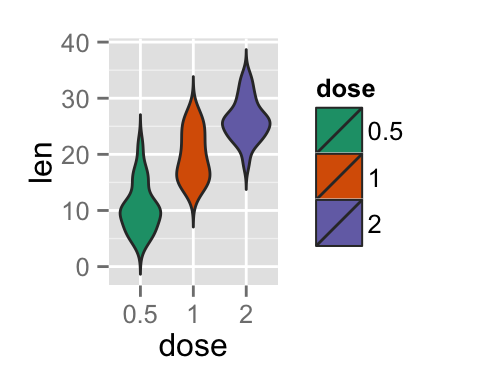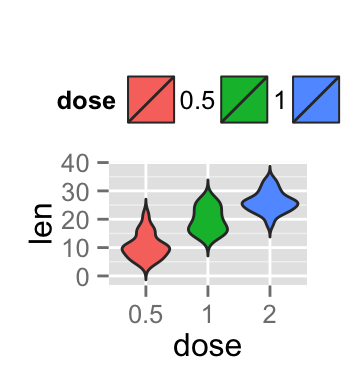# ggplot2 violin plot : Quick start guide - R software and data visualization

This R tutorial describes how to create a violin plot using R software and ggplot2 package.

violin plots are similar to box plots, except that they also show the kernel probability density of the data at different values. Typically, violin plots will include a marker for the median of the data and a box indicating the interquartile range, as in standard box plots.

The function geom_violin() is used to produce a violin plot.# Prepare the data

ToothGrowth data sets are used :

``````# Convert the variable dose from a numeric to a factor variable
ToothGrowth\$dose <- as.factor(ToothGrowth\$dose)
``````##    len supp dose
## 1  4.2   VC  0.5
## 2 11.5   VC  0.5
## 3  7.3   VC  0.5
## 4  5.8   VC  0.5
## 5  6.4   VC  0.5
## 6 10.0   VC  0.5``````

Make sure that the variable dose is converted as a factor variable using the above R script.

# Basic violin plots

``````library(ggplot2)
# Basic violin plot
p <- ggplot(ToothGrowth, aes(x=dose, y=len)) +
geom_violin()
p
# Rotate the violin plot
p + coord_flip()
# Set trim argument to FALSE
ggplot(ToothGrowth, aes(x=dose, y=len)) +
geom_violin(trim=FALSE)``````Note that by default trim = TRUE. In this case, the tails of the violins are trimmed. If FALSE, don’t trim the tails

Choose which items to display :

``p + scale_x_discrete(limits=c("0.5", "2"))``# Add summary statistics on a violin plot

The function stat_summary() can be used to add mean/median points and more on a violin plot.

## Add mean and median points

``````# violin plot with mean points
p + stat_summary(fun.y=mean, geom="point", shape=23, size=2)
# violin plot with median points
p + stat_summary(fun.y=median, geom="point", size=2, color="red")``````A solution is to use the function geom_boxplot :

``p + geom_boxplot(width=0.1)``## Add mean and standard deviation

The function mean_sdl is used. mean_sdl computes the mean plus or minus a constant times the standard deviation.

In the R code below, the constant is specified using the argument mult (mult = 1). By default mult = 2.

The mean +/- SD can be added as a crossbar or a pointrange :

``````p <- ggplot(ToothGrowth, aes(x=dose, y=len)) +
geom_violin(trim=FALSE)
p + stat_summary(fun.data="mean_sdl", mult=1,
geom="crossbar", width=0.2 )
p + stat_summary(fun.data=mean_sdl, mult=1,
geom="pointrange", color="red")``````Note that, you can also define a custom function to produce summary statistics as follow :

``````# Function to produce summary statistics (mean and +/- sd)
data_summary <- function(x) {
m <- mean(x)
ymin <- m-sd(x)
ymax <- m+sd(x)
return(c(y=m,ymin=ymin,ymax=ymax))
}``````

Use a custom summary function :

``p + stat_summary(fun.data=data_summary)``# Violin plot with dots

Dots (or points) can be added to a violin plot using the functions geom_dotplot() or geom_jitter() :

``````# violin plot with dot plot
p + geom_dotplot(binaxis='y', stackdir='center', dotsize=1)
# violin plot with jittered points
# 0.2 : degree of jitter in x direction
p + geom_jitter(shape=16, position=position_jitter(0.2))``````# Change violin plot colors by groups

## Change violin plot line colors

Violin plot line colors can be automatically controlled by the levels of dose :

``````# Change violin plot line colors by groups
p<-ggplot(ToothGrowth, aes(x=dose, y=len, color=dose)) +
geom_violin(trim=FALSE)
p``````It is also possible to change manually violin plot line colors using the functions :

• scale_color_manual() : to use custom colors
• scale_color_brewer() : to use color palettes from RColorBrewer package
• scale_color_grey() : to use grey color palettes
``````# Use custom color palettes
p+scale_color_manual(values=c("#999999", "#E69F00", "#56B4E9"))
# Use brewer color palettes
p+scale_color_brewer(palette="Dark2")
# Use grey scale
p + scale_color_grey() + theme_classic()``````Read more on ggplot2 colors here : ggplot2 colors

## Change violin plot fill colors

In the R code below, the fill colors of the violin plot are automatically controlled by the levels of dose :

``````# Use single color
ggplot(ToothGrowth, aes(x=dose, y=len)) +
geom_violin(trim=FALSE, fill='#A4A4A4', color="darkred")+
geom_boxplot(width=0.1) + theme_minimal()
# Change violin plot colors by groups
p<-ggplot(ToothGrowth, aes(x=dose, y=len, fill=dose)) +
geom_violin(trim=FALSE)
p``````It is also possible to change manually violin plot colors using the functions :

• scale_fill_manual() : to use custom colors
• scale_fill_brewer() : to use color palettes from RColorBrewer package
• scale_fill_grey() : to use grey color palettes
``````# Use custom color palettes
p+scale_fill_manual(values=c("#999999", "#E69F00", "#56B4E9"))
# Use brewer color palettes
p+scale_fill_brewer(palette="Dark2")
# Use grey scale
p + scale_fill_grey() + theme_classic()``````Read more on ggplot2 colors here : ggplot2 colors

# Change the legend position

``````p + theme(legend.position="top")
p + theme(legend.position="bottom")
p + theme(legend.position="none") # Remove legend``````The allowed values for the arguments legend.position are : “left”,“top”, “right”, “bottom”.

Read more on ggplot legends : ggplot2 legend

# Change the order of items in the legend

The function scale_x_discrete can be used to change the order of items to “2”, “0.5”, “1” :

``p + scale_x_discrete(limits=c("2", "0.5", "1"))``# Violin plot with multiple groups

``````# Change violin plot colors by groups
ggplot(ToothGrowth, aes(x=dose, y=len, fill=supp)) +
geom_violin()
# Change the position
p<-ggplot(ToothGrowth, aes(x=dose, y=len, fill=supp)) +
geom_violin(position=position_dodge(1))
p``````Change violin plot colors and add dots :

``````# Add dots
p + geom_dotplot(binaxis='y', stackdir='center',
position=position_dodge(1))
# Change colors
p+scale_fill_manual(values=c("#999999", "#E69F00", "#56B4E9"))``````# Customized violin plots

``````# Basic violin plot
ggplot(ToothGrowth, aes(x=dose, y=len)) +
geom_violin(trim=FALSE, fill="gray")+
labs(title="Plot of length  by dose",x="Dose (mg)", y = "Length")+
geom_boxplot(width=0.1)+
theme_classic()
# Change color by groups
dp <- ggplot(ToothGrowth, aes(x=dose, y=len, fill=dose)) +
geom_violin(trim=FALSE)+
geom_boxplot(width=0.1, fill="white")+
labs(title="Plot of length  by dose",x="Dose (mg)", y = "Length")
dp + theme_classic()``````Change fill colors manually :

``````# Continusous colors
dp + scale_fill_brewer(palette="Blues") + theme_classic()
# Discrete colors
dp + scale_fill_brewer(palette="Dark2") + theme_minimal()
dp + scale_fill_brewer(palette="RdBu") + theme_minimal()``````Read more on ggplot2 colors here : ggplot2 colors

# Infos

This analysis has been performed using R software (ver. 3.1.2) and ggplot2 (ver. 1.0.0)